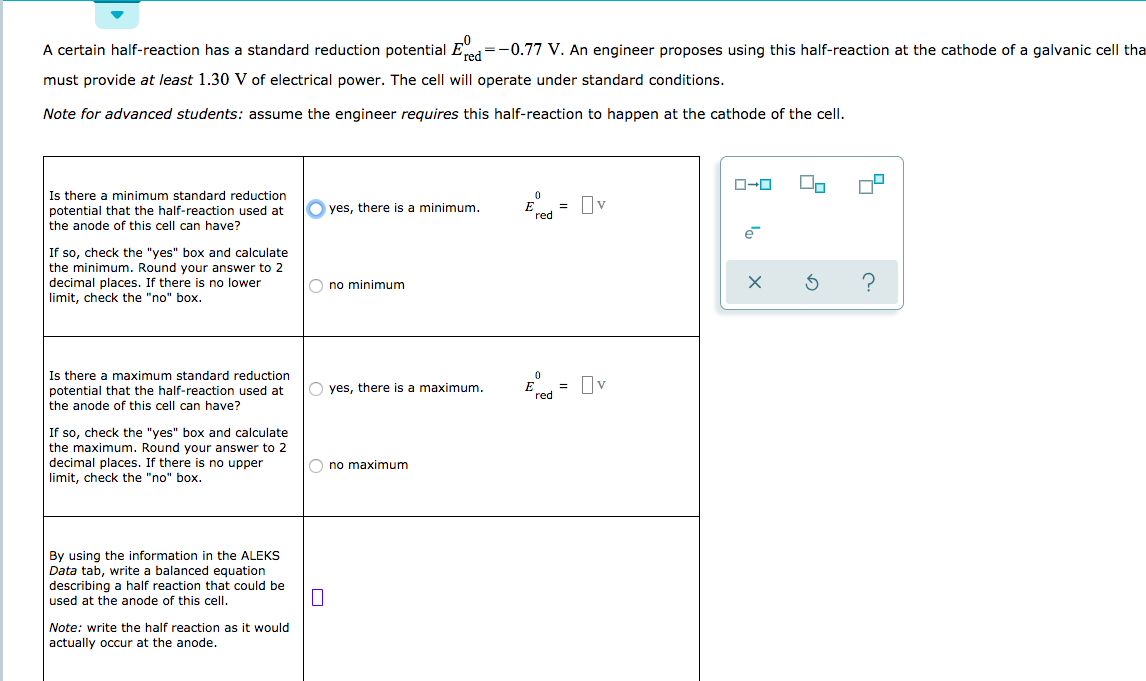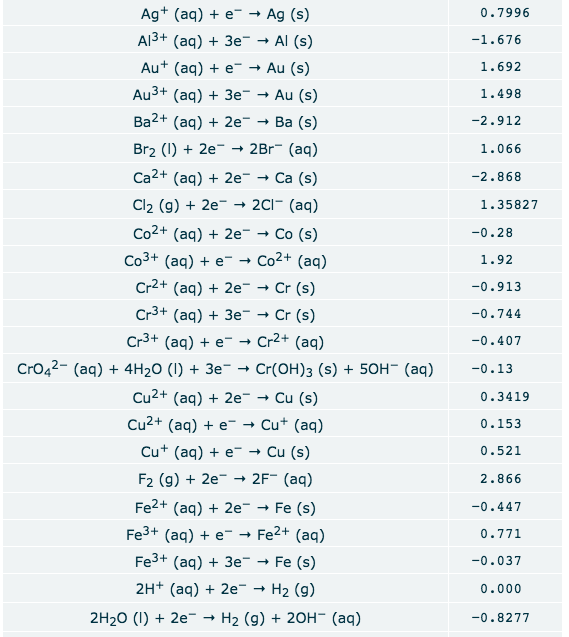# A certain half-reaction has a standard reduction potential E- =-0.77 V. An engineer proposes using this half-reaction at the cathode of a galvanic cell tha must provide at least 1.30 V of electrical power. The cell will operate under standard conditions. Note for advanced students: assume the engineer requires this half-reaction to happen at the cathode of the cell Is there a minimum standard reduction 0 =v yes, there is a minimum. E potential that the half-reaction used at red the anode of this cell can have? If so, check the "yes" box and calculate the minimum. Round your answer to 2 decimal places. If there is no lower limit, check the "no" box X no minimum Is there a maximum standard reduction 0 E red 3 Пv O yes, there is a maximum potential that the half-reaction used at the anode of this cell can have? If so, check the "yes" box and calculate the maximum. Round your answer to 2 mal places. If there is no upper limit, check the "no" box no maximum By using the information in the ALEKS Data tab, write a balanced equation describing a half reaction that could be used at the anode of this cell Note: write the half reaction as it would actually occur at the anode. Ag (aq)e- Ag (s) Al3+ (aq) 3e Al (s) 0.7996 -1.676 Au (aq) e" Au (s) 1.692 Au3+ (aq) 3e Au (s) 1.498 Ba2+ (aq)2e Ba (s) -2.912 Br2 ()2e - 2Br (aq) 1.066 Ca2+ (aq)2e - - Са (s) -2.868 Cl2 (g)2e » 2Cl (aq) 1.35827 Co2+ (aq) 2e Co (s) -0.28 Co3+ (aq) e- Co2+ (aq) 1.92 Cr2+ (aq) 2e Cr (s) -0.913 Cr3+ (ag) 3e Cr (s) -0.744 Cr3+ (aq) e- Cr2+ (aq) -0.407 CrO42- (aq) 4H20 (I) +3e Cr(OH)3 (s) 50H- (aq) -0.13 Cu2+ (aq)2e- Cu (s) 0.3419 Cu2+ (aq) e Cu (aq) 0.153 Cu (aq)e-Cu (s) 0.521 F2 (g)2e 2F (aq) Fe2+ (aq)2e 2.866 Fe (s) -0.447 Fe3+ (aq)e"- Fe2+ (aq) 0.771 Fe3+ (aq) 3e Fe (s) -0.037 2H+ (aq) 2e H2 (g) 0.000 2H20 ()2e- H2 (g) + 20H (aq) -0.8277

Question

If you need the rest of the question let me knowhelp_outlineImage TranscriptioncloseA certain half-reaction has a standard reduction potential E- =-0.77 V. An engineer proposes using this half-reaction at the cathode of a galvanic cell tha must provide at least 1.30 V of electrical power. The cell will operate under standard conditions. Note for advanced students: assume the engineer requires this half-reaction to happen at the cathode of the cell Is there a minimum standard reduction 0 =v yes, there is a minimum. E potential that the half-reaction used at red the anode of this cell can have? If so, check the "yes" box and calculate the minimum. Round your answer to 2 decimal places. If there is no lower limit, check the "no" box X no minimum Is there a maximum standard reduction 0 E red 3 Пv O yes, there is a maximum potential that the half-reaction used at the anode of this cell can have? If so, check the "yes" box and calculate the maximum. Round your answer to 2 mal places. If there is no upper limit, check the "no" box no maximum By using the information in the ALEKS Data tab, write a balanced equation describing a half reaction that could be used at the anode of this cell Note: write the half reaction as it would actually occur at the anode. fullscreenhelp_outlineImage TranscriptioncloseAg (aq)e- Ag (s) Al3+ (aq) 3e Al (s) 0.7996 -1.676 Au (aq) e" Au (s) 1.692 Au3+ (aq) 3e Au (s) 1.498 Ba2+ (aq)2e Ba (s) -2.912 Br2 ()2e - 2Br (aq) 1.066 Ca2+ (aq)2e - - Са (s) -2.868 Cl2 (g)2e » 2Cl (aq) 1.35827 Co2+ (aq) 2e Co (s) -0.28 Co3+ (aq) e- Co2+ (aq) 1.92 Cr2+ (aq) 2e Cr (s) -0.913 Cr3+ (ag) 3e Cr (s) -0.744 Cr3+ (aq) e- Cr2+ (aq) -0.407 CrO42- (aq) 4H20 (I) +3e Cr(OH)3 (s) 50H- (aq) -0.13 Cu2+ (aq)2e- Cu (s) 0.3419 Cu2+ (aq) e Cu (aq) 0.153 Cu (aq)e-Cu (s) 0.521 F2 (g)2e 2F (aq) Fe2+ (aq)2e 2.866 Fe (s) -0.447 Fe3+ (aq)e"- Fe2+ (aq) 0.771 Fe3+ (aq) 3e Fe (s) -0.037 2H+ (aq) 2e H2 (g) 0.000 2H20 ()2e- H2 (g) + 20H (aq) -0.8277 fullscreen

## Expert Answer

### Want to see this answer and more?

Experts are waiting 24/7 to provide step-by-step solutions in as fast as 30 minutes!*

*Response times may vary by subject and question complexity. Median response time is 34 minutes for paid subscribers and may be longer for promotional offers.
Tagged in
Science
Chemistry© 2021 bartleby. All Rights Reserved.